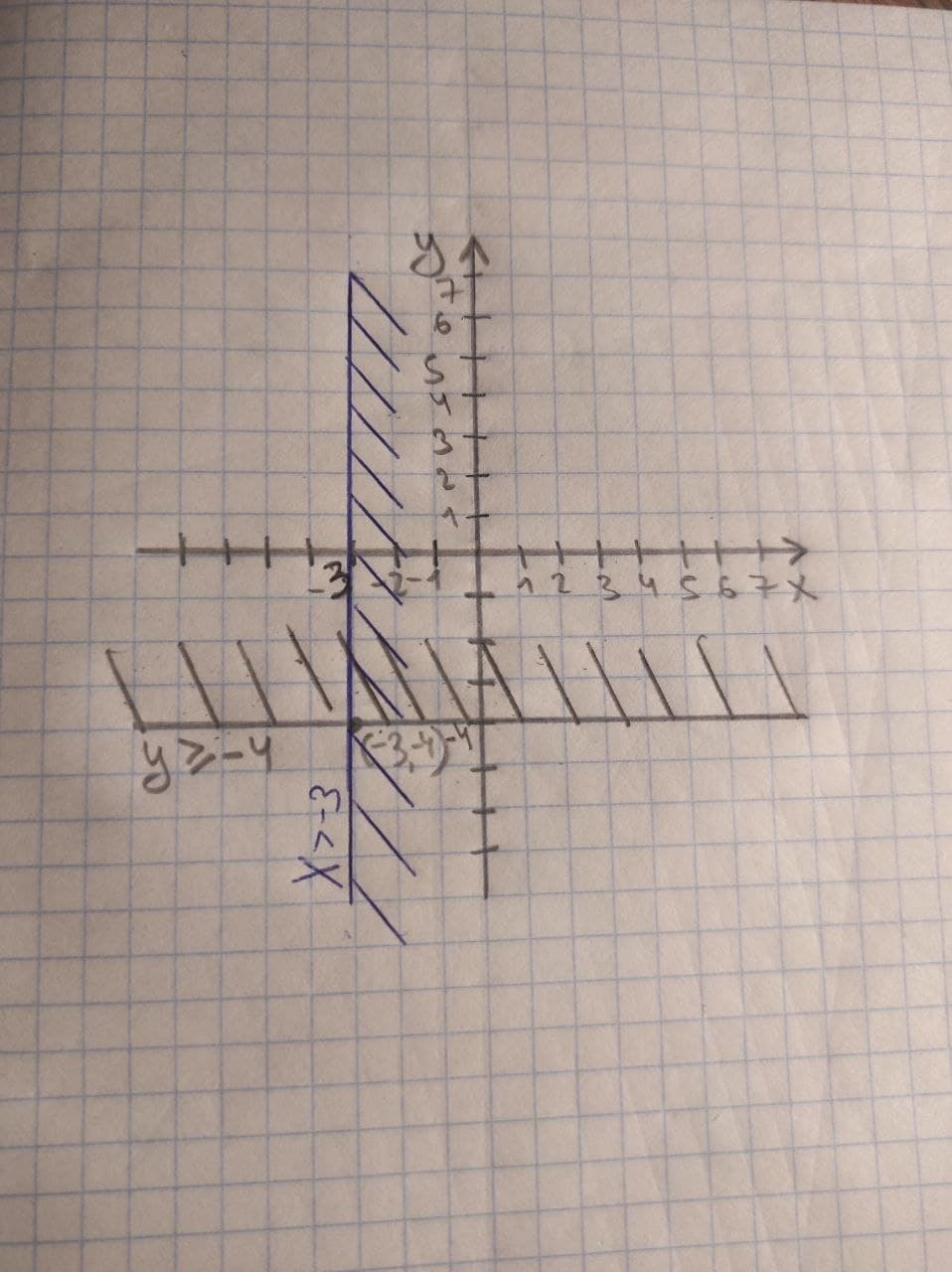# Graph the solution set for the system of linear inequalities x>-3y>=-4generals336 2021-02-25 Answered

Graph the solution set for the system of linear inequalities
$x>-3$
$y\ge -4$

You can still ask an expert for help

## Want to know more about Inequalities systems and graphs?

• Questions are typically answered in as fast as 30 minutes

Solve your problem for the price of one coffee

• Math expert for every subject
• Pay only if we can solve itCaren
The solution set:
$x\in \left(-3,\mathrm{\infty }\right),$
$y\in \left[-4,\mathrm{\infty }\right)$
The graph of solution set: